Courses

# Test: General Organic Chemistry Level - 3

## 30 Questions MCQ Test Organic Chemistry | Test: General Organic Chemistry Level - 3

Description
This mock test of Test: General Organic Chemistry Level - 3 for Chemistry helps you for every Chemistry entrance exam. This contains 30 Multiple Choice Questions for Chemistry Test: General Organic Chemistry Level - 3 (mcq) to study with solutions a complete question bank. The solved questions answers in this Test: General Organic Chemistry Level - 3 quiz give you a good mix of easy questions and tough questions. Chemistry students definitely take this Test: General Organic Chemistry Level - 3 exercise for a better result in the exam. You can find other Test: General Organic Chemistry Level - 3 extra questions, long questions & short questions for Chemistry on EduRev as well by searching above.
*Multiple options can be correct
QUESTION: 1

### Which of the following compounds is/are aromatic?

Solution:
*Multiple options can be correct
QUESTION: 2

### Which of the following has (have) (4n + 2) π-electrons but not aromatic?

Solution:

A and D have 4n+2 electrons but are not aromatic as they are non-planar.

*Multiple options can be correct
QUESTION: 3

### Which of the given statement(s) about N, O, P and Q with respect to M is (are) correct?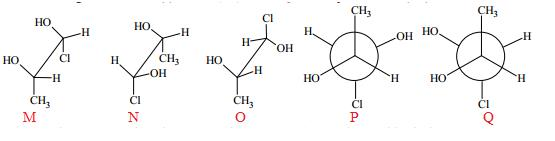Solution:
QUESTION: 4

A compound does not shows aromaticity when it have:

Solution:

Explanation : Aromatic π-sextets are defined as six π-electrons localized in a single benzene-like ring separated from adjacent rings by formal CC single bonds.Therefore, Aromatic sextet compound does not shows aromaticity.

*Multiple options can be correct
QUESTION: 5

Which of the system is /are aromatic?

Solution:
*Multiple options can be correct
QUESTION: 6

Select the compounds which is/are anti-aromatic?

Solution:

For aromatic-compound should be cyclic, sp2 hybridised, planar, (4n+2)πe- for anti-aromatic-compound should be cyclic, sp2 hybridised, planar, (4n)πe-
Hence compound A and D has anti-aromatic character.

*Multiple options can be correct
QUESTION: 7

Which of the following compounds is expected to undergo faster electrophilic subst itution than unsubstituted benzene?

Solution:
*Multiple options can be correct
QUESTION: 8

Which of the following compounds are aromatic?

Solution: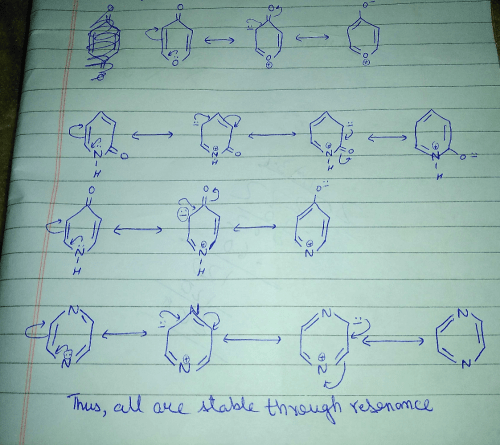*Multiple options can be correct
QUESTION: 9

Which of the following compounds is/are aromatic?

Solution:

Explanation : The term aromaticity is used to describe a cyclic, planar molecule with a ring of resonance bonds that exhibits more stability than other geometric or connective arrangements with the same set of atoms.

Only the peripheral pie electrons are considered! doesn't matter whether that central carbon is sp2 or not.

A,B have all sp2 carbon atoms and follow (4n+2)pie electron rule so are aromatic.

*Multiple options can be correct
QUESTION: 10

Which of the following compounds are non-aromatic?

Solution:

C and D are non-aromatic because C is nonplanar and D is having one carbon without hybridization.

*Multiple options can be correct
QUESTION: 11

Which of the following is/are aromatic?

Solution:
*Multiple options can be correct
QUESTION: 12

Which of the following molecules, in pure form, is (are) unstable at room temperature:

Solution:

Explanation : (B) Cyclobutadiene follows Huckel's criteria for anti-aromaticity [(4n)−π electrons],  hence unstable.

(C) Follows Huckel's criteria for anti-aromaticity [(4n)−π electrons], hence, unstable.

QUESTION: 13

Hybridizat ions of the atoms indicated with the asterisk (*) in the fo llowing compounds sequent ially are: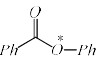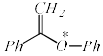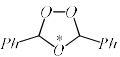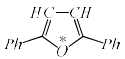Solution:

Explanation : In 3rd compound delocalisation of electron is not possible so hybridization = 2sigma + 2loan pair of electron

= sp3.

In 1st,2nd,4th compound one loan pair of oxygen can delocalise so these electron are count as pi electron there for hybridization of oxygen = 2sigma + 1loan pair of electron

= sp2

*Multiple options can be correct
QUESTION: 14

Which of the following species is/are aromatic in nature?

Solution:
*Multiple options can be correct
QUESTION: 15

Which of the following species is/are aromatic in nature?

Solution:
QUESTION: 16

What is the product of the following reaction?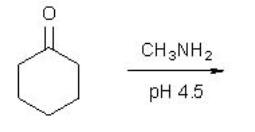Solution:
QUESTION: 17

The correct order dipole moments (μ) of the following compounds is:

(1) CH3CH2CH2CHO
(2) CH3CH = CHCHO
(3) CH3CH2CH = CH2

Solution:

(1) CH3CH2CH2CHO - 2.720 Debye
(2) CH3CH = CHCHO - 3.720 Debye
(3) CH3CH2CH = CH2  - 0.359 Debye

QUESTION: 18

The value of ‘n’ for the following molecule according to Hucke’s rule is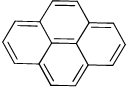Solution:

Explanation : According to Hucckel’s rule

We take pi bonds only on the periphery and do not count one not on boundaries or periphery.

4n + 2 = 14

4n = 12

n = 3

QUESTION: 19

The correct statement describing the relationship between following structures is: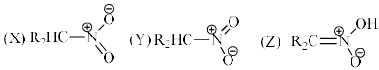Solution:
QUESTION: 20

The correct order of acidity among the following structures is: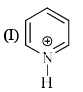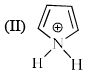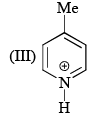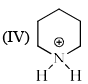Solution:

Pyrrolidine is strongest base, thus having the weakest conjugate acid so, option B correct.

QUESTION: 21

Arrange the following in the correct order of acidity of the hydrogen indicated in bold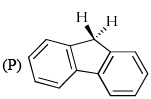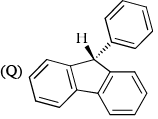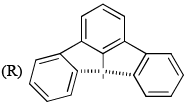Solution:

Explanation : In R negative charge delocalised into two rings make them aromatic which are further stabilized by 3 benzene rings. while in P and Q negative charge delocalised to one ring make it aromatic which are further stabilized by two benzene rings. but Q have extra resonance in compare to P

QUESTION: 22

Which of the following are aromatic?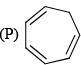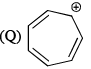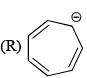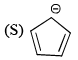Solution:
QUESTION: 23

The species/compounds that are aromatic among the following are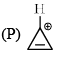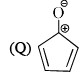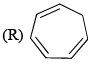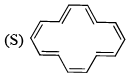Solution:

By considering Huckel’s Rule P and S are correct.

Hence D is the correct answer.

QUESTION: 24

The homolytic breaking of the Ca— Cb bond is easiest in:

Solution:
QUESTION: 25

Which of the following represent the given monde of hydridisation sp2— sp2—sp—sp from left to righ?

Solution:

H2C=CH−C≡N
H2C⇒sp2
CH⇒sp2
C⇒sp
N⇒sp
Hence (a) is the correct answer.

QUESTION: 26

Arrange the following free radicals in their stability order: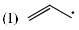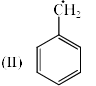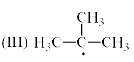Solution:

QUESTION: 27

The correct order of pKa for the following compounds is: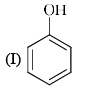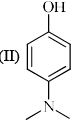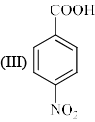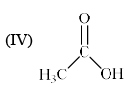Solution:
QUESTION: 28

The correct statement (s) about the following compounds is: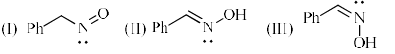Solution: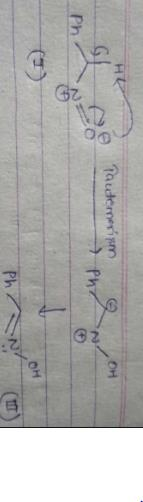QUESTION: 29

Arrange the pKas of the hydroxy groups of methanol, trifluoroacetic acid, phenol and benzyl alcohol in ascending order:

Solution:
QUESTION: 30

Among the resonance forms given below, the one which contributes most to the stability of azulene is

Solution: